CBSE Sample Papers for Class 12 Chemistry Paper 6 are part of CBSE Sample Papers for Class 12 Chemistry. Here we have given CBSE Sample Papers for Class 12 Chemistry Paper 6.

## CBSE Sample Papers for Class 12 Chemistry Paper 6

 Board CBSE Class XII Subject Chemistry Sample Paper Set Paper 6 Category CBSE Sample Papers

Students who are going to appear for CBSE Class 12 Examinations are advised to practice the CBSE sample papers given here which is designed as per the latest Syllabus and marking scheme as prescribed by the CBSE is given here. Paper 6 of Solved CBSE Sample Paper for Class 12 Chemistry is given below with free PDF download solutions.

Time Allowed : 3 Hours
Max. Marks : 70

General Instructions

• All questions are compulsory.
• Question number 1 to 5 are very short answer questions and carry 1 mark each.
• Question number 6 to 10 are short answer questions and carry 2 marks each.
• Question number 11 to 22 are also short answer questions and carry 3 marks each.
• Question number 23 is a value based question and carry 4 marks.
• Question number 24 to 26 are long answer questions and carry 5 marks each.
• Use log table, if necessary. Use of calculators is not allowed.

Questions

Question 1.
Which of the 3d series element is not considered as a transition element?
OR
Which transition element of group XII shows two different oxidation states?

Question 2.
Write IUPAC name of the following compound:
CH3COCH2-CH = CH-CHO

Question 3.
For a reaction in 10 minutes concentration of reactant reduced from 0.12 µ to 0.06 µ and in next 10 minutes it becomes 0.03 µ. Find order of reaction for this reaction.

Question 4.
Draw structure of the methyl hemiacetal of formaldehyde.

Question 5.
Why does physisorption decrease with the increase of temperature?

Question 6.
Complete the following reactions:

1. Pb(NO3)2 $$\underrightarrow { Heat }$$
2. XeOF4 + H2O →

OR

1. P4 + HNO3 (Conc.) →
2. PCl3 + 3H2O →

Question 7.
Define the following terms:

1. Mole fraction
2. Reverse osmosis

Question 8.
Draw the structures of the following:
(a) (HPO3)3
(b) XeF2

Question 9.
Calculate the equilibrium constant of the following reaction at 298 K:
Cu(s) + 2Ag+(aq) → Cu2+(aq) + 2Ag(s), ECell° = 0.46 V

Question 10.
Write the equation involved in the following reaction

1. Sandmeyer’s reaction
2. Hinsberg’s reagent with 1° amine

Question 11.
Henry’s law constant for the molality of CH4 in benzene at 298 K is 0.562 k atm. Calculate the solubility of methane in benzene at 298 K under 760 mmHg.

Question 12.
Resistance of a conductivity cell filled with 0.1 M KCl solution is 100 Ω. If the resistance of the same cell when filled with 0.02 M KCl solution is 520 Ω, calculate the conductivity, molar conductivity of the cell having 0.02 M KCl solution. (The conductivity of 0.1 M KCl solution is 1.29 S/m).

Question 13.
(a) Using VBT predict the geometry and magnetic behaviour of [CoF6]3-.
(b) Draw the structures of geometrical isomers of [Fe(CN)4(NH3)2].

Question 14.
Define lyophilic and lyophobic colloids. Give one example of each type. Which one of these two types of sol is easily coagulated and why?

Question 15.
The following data were obtained during the first order thermal decomposition of N2Os(g) at constant volume:Calculate the rate constant.

Question 16.
Among the isomeric alkanes of molecular formula C5H12, identify the one that on photochemical chlorination yields:
(a) A single monochloride which does not gives elimination reaction.
(b) Three isomeric monochloride
(c) Four isomeric monochloride

Question 17.
State briefly the principle involved in the following processes:

1. Froth flotation process
2. Chromatography
3. Magnetic separation

Question 18.
Give the structures of A, B and C in the following reactions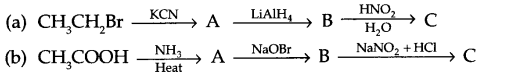Question 19.
Write the structures of the monomers used for the following polymer:
(a) PVC
(b) Nylon-6
(c) Teflon

Question 20.
Give reason for the following:

1. Aspartame is limited to cold food only.
2. Allergic action is caused by histamine.
3. Medicines should not be taken without consulting doctors.

Question 21.
Assign suitable reason for the following:
(a) Bond angle of NO2 is less than NO+2.
(b) Sulphur in vapour phase shows paramagnetic behaviour.
(c) Noble gases have very low Boiling point.

Question 22.
Give reasons for:

1. Aldehydes are more reactive towards addition nucleophilic reaction as compared to ketones.
2. Phenol is less acidic than carboxylic acid though it has more number of resonating structures.
3. During the preparation of ester from a carboxylic acid and an alcohol in the presence of an acid catalyst, ester should be distilled off as soon as it forms.

Question 23.
Sapna a domestic helper of Mrs. Sheela had a wound on her arm but she was not caring for it. Sheela applied dettol on her wound and asked her to show it to a doctor if it does not heal.

1. What type of drugs are applied on wounds to prevent the growth of micro-organisms?
2. Name the components of dettol.
3. Name two other chemical substances of this category.
4. What values are associated with Mrs. Sheela?

Question 24.

1. Name a member of the lanthanoid series which is well known to exhibit +4 oxidation state.
2. Actinoid contraction is greater from element to element them lanthanoid contraction. Give reason.
1. Complete the following chemical reactions
1. MnO4 + I (aq) $$\underrightarrow { { OH }^{ – } }$$
2. NO2 + MnO4 + H+
3. Cr2O2-7 + Fe2+ + H+

OR
On the basis of lanthanoid contraction, explain the following:

1. Nature of bonding in La2O3 and Lu2O3.
2. Trends in the stability of oxosalts of lanthanoid as from La to Lu.
3. Stability of the complexes of lanthanoids.
4. Radii of 4d and 5d block elements.
5. Trends in acidic character of lanthanoid oxides.

Question 25.
(a) A compound is formed by two elements M & N. The element N form ccp array and atoms
of the element M occupy 1/3 rd the tetrahedral void. What is the formula of the compound?
(b) An element has a ccp structure with radii of atom 141.4 pm. The density of the element is 8 g/cm3. How many atoms are present in 220 g of this element?
OR
(a) Describe the two main types of semiconductors and contrast their conduction mechanism.
(b) An element with molar mass 2.7 × 10-2 kg/mol forms a cubic unit cell with edge length 405 pm. It its density is 2.7 kg/m3, what is the nature of the cubic unit cell?

Question 26.
(a) Give the mechanism for formation of ethoxyethane from the ethanol.
(b) Distinguish the following pairs:
(i) Ethanol and propan-l-ol
(ii) Anisole and phenol
(iii) Ethoxyethane and ethanol

OR
(a) Arrange according to the given instruction:
(i) Methoxy phenol, o-chlorophenol and o-nitrophenol (Acidic strength)
(ii) Ethanol, propane, methoxy methane (Boiling point)
(iii) Butan-1-ol, 2-methyl propan-2-ol, Butan-2-ol (elimination reaction)
(b) (i) Phenol is more acidic than ethanol. Why?
(ii) Write the chemical reaction for the preparation of phenol from cumene

Zinc (Zn) element of 3d-series is not considered as a transition element.
OR
Hg (Hg+ and Hg2+)

5-oxohex-2-enal

Since in every 10-minutes concentration becomes half, so it is a first order reaction.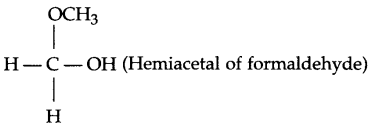Physiosorption is an exothermic process. Thus with increase in temperature, the tendency of physiosorbed molecules to be retained on the surface of adsorbent decreases.

1. 2Pb(NO3)2 $$\underrightarrow { Heat }$$ 4NO2 + 2PbO
2. XeOF4 + H2O → XeO2F2 + 2HF

OR

1. P4 + 20HNO3 (Conc.) → 4H3PO4 + 20NO2 + 4H2O
2. PCl3 + 3H2O → H3PO3 + 3HCl

1. Mole fraction : It is fraction of a component present in each mole of solution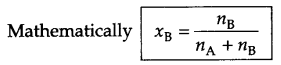2. Reverse osmosis : It is the movement of pure solvent molecules from solution through semi-permeable membrane on application of pressure greater than osmotic pressure to the higher concentration side.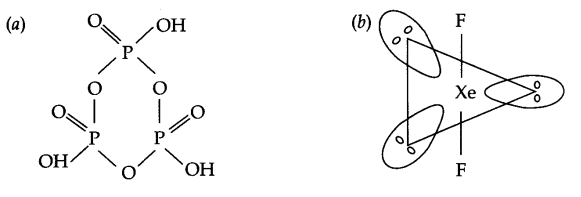Cu(s) + 2Ag+(aq) → Cu2+(aq) + 2Ag (s) n = 2
Since ΔG° = – nFECell° = – 2.303 RT log Kc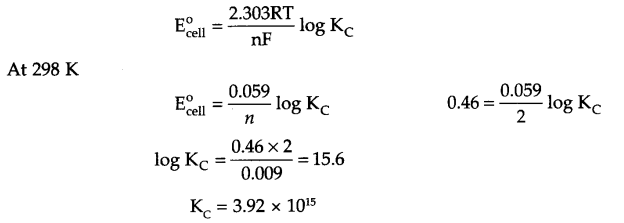1. Sandmeyer’s reaction : Freshly prepared diazonium salt on mixing with cuprous chloride or bromide gives chloro or bromo benzene respectively. Such a reaction is known as Sandmeyer’s Reaction.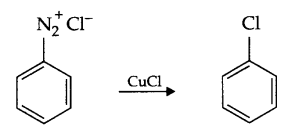2. Hinsberg’s reagent with 1° amine :
Benzene sulphonyl chloride is known as Hinsberg’s reagent: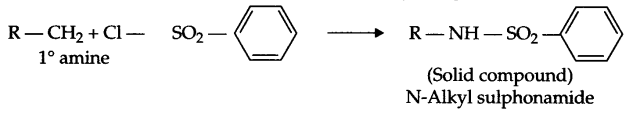KH = 0.562 k atm = 0.562 × 103 atm
Pressure of gas P(g) = 760 mm Hg = 1 atm
from Henry’s lawSince,
Cell constant (G*) = Conductivity (K) × resistance (R)
= 1.29 S/m × 100 Ω = 129 m-1 = 1.29 cm-1
∴ K for 0.02 M KCl solution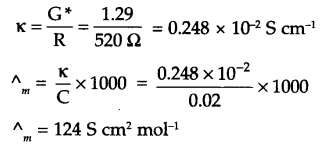(a) According to V.B.T the electronic arrangement of [COF6]3-.
Co(27) → [Ar] 3d74s2
Co3+ → [Ar] 3d6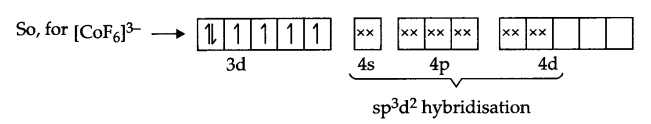It’s Geometry is octahedral, and it is paramagnetic in nature.
(b) Geometrical isomers of [Fe(CN)4 (NH3)2] :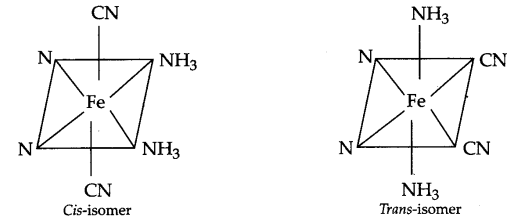• Lyophilic colloids : In these, there are attracting forces acting between particles of dispersion medium and dispersed phase and hence these are known as lyophilic colloids. These are reversible in nature.
• Lyphobic Colloids : There is no interaction or have very little interactions between the particles of dispersed phase and dispersion medium, i.e. these are solvent repelling colloids. Hence they known lyophobic colloids and they are irreversible in nature.
Example of Lyophilic colloids -Starch sol, gum, gelatine.
Lyophilic colloids -Arsenic sulphide sol, Au sol, sulphur sol etc.
Lyophobic sols can easily get coagulated.
• Reason : Due to very less interactions between dispersed phase and dispersion medium, coagulation takes place easily.

If pressure of N2O5 (g) decrease by 2x atm, thenTotal pressure Pt = 0.5 + x = 0.512
So x = 0.012
Pressure of N2O5 at time t = 100 s is 0.5 – 2x
i.e. Pt = 0.5 – 2 × 0.012 0.476 atm
Since for a first order reaction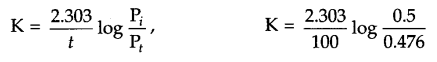K = 4.98 × 10-4 s-1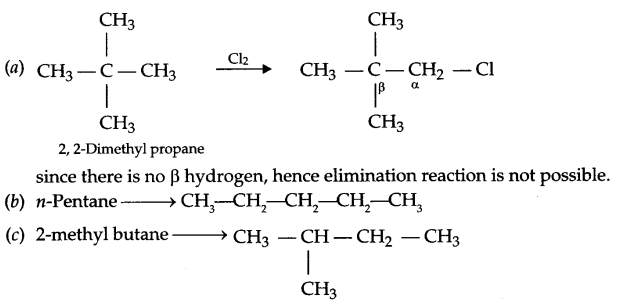1. Froth floatation process : Different wettability of ore and gangue particles is the principle involved.
2. Chromatography : The principle involved in this process is different adsorbability of various components of a mixture.
3. Magnetic separation : This is based on the principle of different magnetic properties of the ore components. If either the ore or the gangue particles is capable of being attracted by a magnetic field, then such separation can be carried out.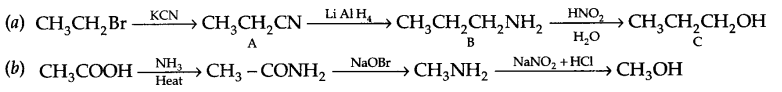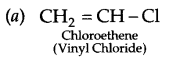1. Aspartame gets decomposed at cooking temperature, hence limited to cold food only.
2. When foreign proteins, flower pollens or any disease causing pathogens attached to mast cell it produces histamine for allergic action to act against these foreign particles.
3. Drugs are the chemicals which produces a biological response (pharmacological effect) even when taken in very small dose and may prove fatal. Doctors are aware of all sorts of useful and ill effects of such drugs. Therefore, doctors must be consulted before taking any medicine.(b) In vapour phase, sulphur exists as S2 molecule like O2 and posses impaired electrons in antibonding molecular orbitals (π*3Px and π*3Py).
(c) There is weak vander wall’s forces exist among noble gas molecules, hence they have low boiling points.

1. There is more +I-effect from the two alkyl group of ketones, hence carboxyl carbon has less positive charge and so it will be less reactive than an aldehyde.
2. Phenoxide ion has more number of resonating structures but the negative charge lies on less electronegative carbon atoms, hence contribution of such structures is negligible as compared to the resonating structure of carboxylate ion where negative charge lies on the oxygen atom. So the contribution of resonance structures is much more in case of carboxylate ion.
3. Esterification reaction is a reversible reaction, hence ester is distilled off as soon as it is formed.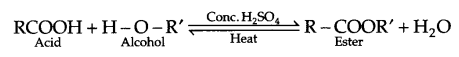1. Antiseptics
2. Chloroxylenol, isopropanol, castor oil, soap and pine oil.
3. Iodoform, Tincture iodine
4. Care for all human beings, knowledge of science, concern for maid servant.

1. Cerium (Ce) (others Gd, Ho, Dy etc)
2. 5f orbital has poorer shielding than 4f orbital, as a result element to element actinoid contraction is greater than lanthanoid contraction.
1. MnO4 + I $$\underrightarrow { { OH }^{ – } }$$ MnO2 + IO3
2. NO2 + MnO4 + H+ → NO3 + Mn2+
3. Cr2O2-7 + Fe2+ + H+ → Cr3+ + Fe3+ + H2O

OR

1. La2Ois ionic because ‘La’ has largest atomic size and largest ionisation enthalpy among the lanthanoids, whereas Lu2O3 is covalent because Lu has smaller size and higher ionisation enthalpy.
2. As the size decreases from La to Lu, therefore the stability of oxo-cations also decreases.
3. The stability of complexes increases as the size of lanthanoids decreases.
4. Radii of 4d and 5d elements are almost same due to lanthanoid contraction.
5. Acidic character of oxides of lanthanoids increases from La to Lu because metallic character decreases due to decrease in atomic size and increase in ionisation enthalpy.

(a) Since element N is in CCP array there will be 4 ‘N’ atoms and 8 tetrahedral voids, out of which 1/3 is occupied by m atoms, hence formula of the compound:
M4×$$\frac { 1 }{ 4 }$$ N3 ⇒ M2N3 is the formula for the given compound.
(b) From question:
Z = 4 for ccp arrangement
radii = 141.4 pm, d = 8 g/cm3
mass of sample = 220 g
No. of atoms in sample (n) = ?OR
(a) Two main types of semiconductors are:
(i) n-type semiconductor
(ii) p-type semiconductor
n-type semiconductors are formed when an element of higher valency is doped in element of lower valency, e.g. Si is doped with Arsenic (As). The extra electron not involved in covalent bond formation increases the conductivity of doped element, p-type semiconductors are formed when element of higher valency is doped with element of lower valency, e.g. Si is doped with Gallium (Ga). It leads to the formation of holes or electron vacancy in doped element.

Conduction mechanism :
n-type semiconductors-Conduction is due to delocalisation of free electrons in the element lattice.
p-type semiconductors-Conduction is due to movement of holes in doped element lattice.
(b) From the given information
Molar mass (M) = 2.7 × 10-2 g mol-1 = 27 g/mol
Edge length (a) = 405 pm = 405 × 10-10 cm
density (d) = 2.7 × 103 kg/m3 = 2.7 g/cm3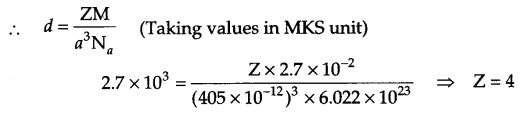Alternately: in CGS unit
Z = 4(b) (i)

 Reagent Ethanol Propan-1-ol NaOH + I2 (heat) Yellow ppt of CHI3 forms No reaction for yellow ppt formation

(ii)

 Reagent Anisole Phenol Neutral FeCl3 No colour change Dark green,grey-violet coloured complex will form

(iii)

 Reagent Ethoxy ethane Ethanol Na metal No H2 gas formation Bubbles of H2 gas will come out.

OR(ii) Propane < methoxy methane < ethanol
(iii) Butan-1-ol < Butan-2-ol < 2-methyl propan-2-ol
(b) (i) Phenoxide ion is resonance stablised, hence phenol is more acidic than ethanol.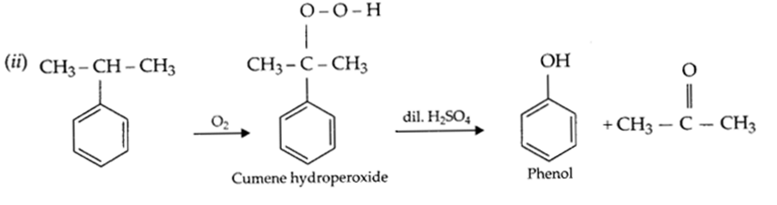We hope the CBSE Sample Papers for Class 12 Chemistry Paper 6 help you. If you have any query regarding CBSE Sample Papers for Class 12 Chemistry Paper 6, drop a comment below and we will get back to you at the earliest.